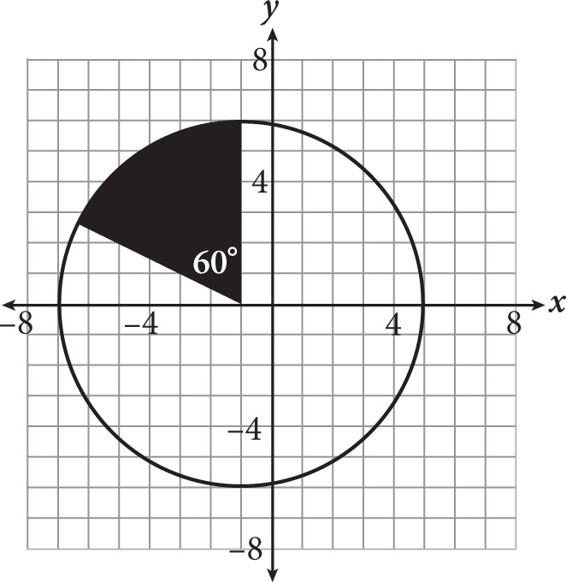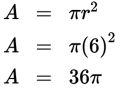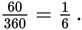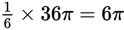# SAT Math Multiple Choice Question 263: Answer and Explanation

### Test Information

Question: 263

8.What is the area of the shaded sector of the circle shown in the figure above?

• A. 2π
• B. 6π
• C. 12π
• D. 36π

Explanation:

B

Difficulty: Medium

Category: Additional Topics in Math / Geometry

Strategic Advice: The area of a sector is equal to the area of the circle times the fraction of the circle represented by the sector.

Getting to the Answer: Start by finding the area of the whole circle: The diameter of the circle extends along the x-axis from -7 to 5, which is 12 units, which means the radius is 6. Substitute this into the area formula:There are 360 degrees in a whole circle, so the fraction of the circle represented by the sector isThe area of the sector issquare units.